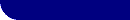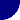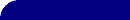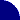# Capacitance Value from CPE: A CalculatorRESOURCES > EIS > CPE > CALCULATING C > CALCULATOR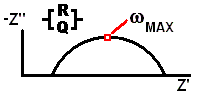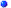This calculator can be used to calculate the "true" capacitance from a depressed semicircle model ( a Constant Phase Element in parallel with a resistor ). If your data has been fit to a depressed semicircle, locate the frequency at the top of the semicircle, and enter omegamax, Qo, and the exponent, n.

Press [Convert...] to update the value of the capacitance.

 Enter Omegamax, CPE parameters: omegamax Hz rad/s Qo S mS uS nS pS s-n n (exponent) 1.0 => n > 0.0 n value should be > 0.75 for reasonable confidence in C values Capacitance F mF uF nF pF Press "Convert..." after you change Capacitance unit.

Uses JavaScript. You must have a JavaScript capable
browser, and have scripting enabled.

 The equation used by this calculator is:  C = Qo * (omegamax)(n-1) The equation for the constant phase element assumed by this calculator is: Z = 1 / [ Qo * ( j *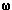)n ]More About EIS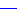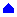REFERENCE(1) "Concerning the Conversion of the Constant Phase Element Parameter Yo into a Capacitance," CS Hsu, F Mansfeld, Corrosion, 57(2001) 747.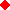Did you find this calculator useful? We'd like to KNOW !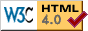© Copyright 2006-2009 Research Solutions & Resources LLC.  All rights reserved.